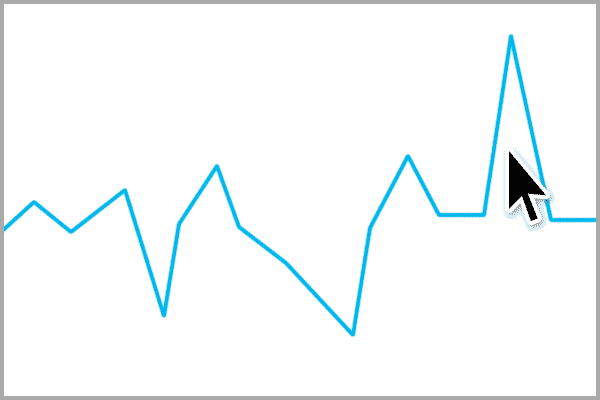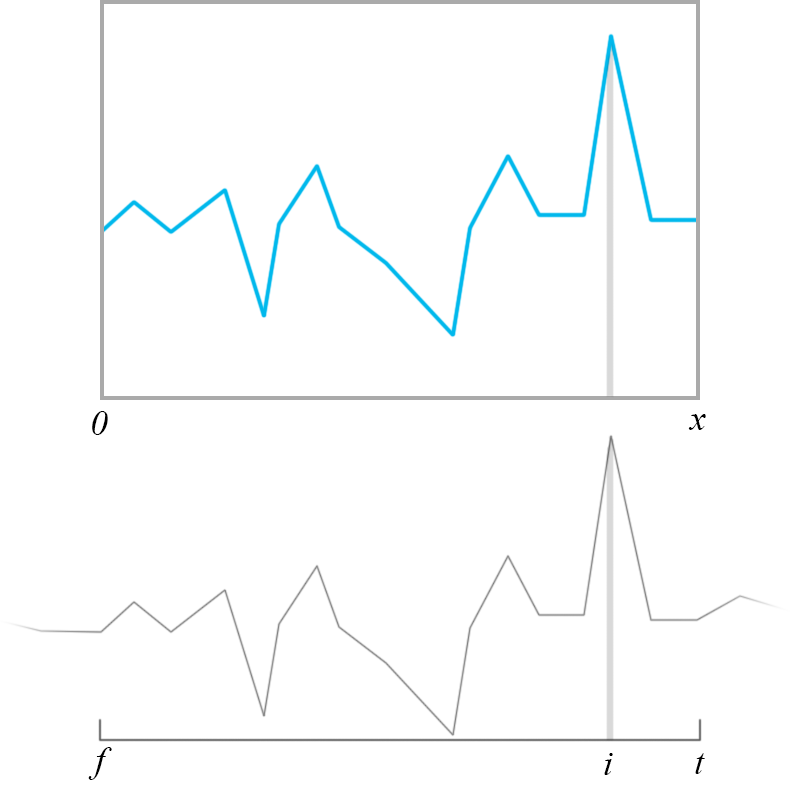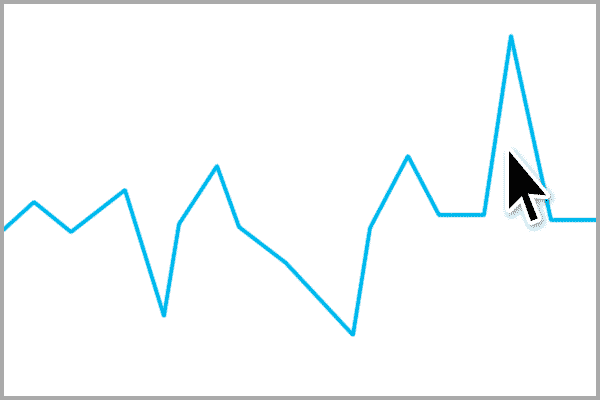https://developer.mozilla.org/en-US/docs/Web/Events/wheel错误缩放示例

（此处有一个丑得不好意思出场的方程……其实我已经找不到了，大概思路是鼠标指针前后加加减减。）映射关系示意图

$$s=\frac{x}{t-f}$$

$$(i-f)\cdot s=(i-{f}')\cdot {s}'$$

$$s={s}'\cdot r$$

$${f}'=i-i\cdot r+f\cdot r$$

$${t}'=i-i\cdot r+t\cdot r$$

$${f}'=f+(1-r)\cdot \frac{t-f}{x}\cdot x_{0}$$

$${t}'=t-(1-r)\cdot \frac{t-f}{x}\cdot (x-x_{0})$$

let tmp = (1 - ratio) * (t - f) / canvasWidth;
let left = f + tmp * (event.offsetX);
let right = t - tmp * (canvasWidth - event.offsetX);正确缩放示例